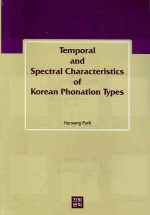> 상세정보

# 상세정보## Temporal and Spectral Characteristics of Korean Phonation Types (2회 대출)

자료유형
단행본
개인저자
Park, Han Sang
서명 / 저자사항
Temporal and Spectral Characteristics of Korean Phonation Types / Park, Han Sang.
발행사항
서울 :   한빛문화 ,   2005.
형태사항
xxvi, 253 p. : 삽도 ; 23cm.
ISBN
8991432085
 000 00576namccc200193 k 4500 001 000045220620 005 20100806112035 007 ta 008 060105s2005 ulka 000a eng 020 ▼a 8991432085 ▼g 93740: ▼c \20,000 040 ▼a 244002 ▼c 244002 ▼d 244002 082 0 4 ▼a 414 ▼2 22 090 ▼a 414 ▼b 2005a 100 1 ▼a Park, Han Sang 245 1 0 ▼a Temporal and Spectral Characteristics of Korean Phonation Types / ▼d Park, Han Sang. 260 ▼a 서울 : ▼b 한빛문화 , ▼c 2005. 300 ▼a xxvi, 253 p. : ▼b 삽도 ; ▼c 23cm. 900 1 ▼a 박한상

### 소장정보

No. 소장처 청구기호 등록번호 도서상태 반납예정일 예약 서비스
No. 1 소장처 청구기호 414 2005a 등록번호 151192092 도서상태 대출가능 반납예정일 예약 서비스

### 컨텐츠정보

#### 목차

```
목차
List of Tables = xix
List of Figures = xxii
Chapter 1 Introduction = 1
1.1 Objectives of the present study = 1
1.2 Significance of the present study = 3
1.3 Organization of the present study = 3
Chapter 2 Phonation Type: Objects and Methods = 7
2.1 Phonation type, consonant type, and voice quality = 7
2.2 Linguistic use of phonation types = 8
2.3 Typology of consonant types = 9
2.4 Phonetic characteristics of voice qualities = 11
2.5 Studies of phonation types = 13
2.6 Acoustic measures of phonation types = 16
2.6.1 Open quotient = 16
2.6.2 Spectral tilt = 19
2.6.3 The first formant bandwidth(B1) = 22
2.7 Summary = 26
Chapter 3 An Acoustic Theory of Vocal Source = 27
3.1 Introductory remarks = 27
3.2 Source Filter Theory = 27
3.3 An acoustic theory of vocal source = 29
3.3.1 Extension of the Source Filter Theory = 29
3.3.2 The radiation characteristic = 31
3.3.3 The source rate = 32
3.3.4 Phonation type index k = 34
3.3.5 A general term for harmonics = 37
3.3.6 Two methods of obtaining phonation type index k = 39
3.3.6.1 The linear regression method = 39
3.3.6.2 The first differencing method = 40
3.4 Summary = 41
Chapter 4 Studies of Korean Phonation Types = 45
4.1 Consonant types of Korean obstruents = 45
4.2 Studies of Korean phonation types = 53
4.2.1 Perceptual studies of Korean phonation types = 53
4.2.2 Production studies of Korean phonation types = 57
4.2.2.1 Physiological aspects of Korean phonation types = 57
4.2.2.1.1 Laryngeal muscle movements = 57
4.2.2.1.2 Glottal width = 60
4.2.2.2 Aerodynamic aspects of Korean phonation types = 61
4.2.2.3 Acoustic aspects of Korean phonation types = 63
4.2.2.3.1 Temporal characteristics of Korean phonation types = 63
4.2.2.3.2 Spectral characteristics of Korean phonation types = 64
4.3 Summary = 67
Chapter 5 Temporal Characteristics of Korean Phonation Types = 73
5.1 Introductory remarks = 73
5.2 Methods = 81
5.2.1 Subjects = 81
5.2.2 Materials = 81
5.2.3 Recording and Digitization = 82
5.2.4 Measurements = 83
5.3 Results = 87
5.3.1 The utterance-initial CV sequence(#CV) = 87
5.3.2 The non-utterance-initial VCV sequence(VCV) = 99
5.3.3 Comparison of the two positions #CV and VCV = 115
5.4 Summary and discussion = 120
5.5 Concluding remarks = 122
Chapter 6 Spectral Characteristics of Korean Phonation Types = 125
6.1 Introductory remarks = 125
6.2 Methods = 125
6.2.1 Measurements = 125
6.2.2 Data processing = 128
6.2.3 Computation of the acoustic measures of phonation types = 133
6.2.3.1 Previous acoustic measures of open quotient = 133
6.2.3.2 Previous measures of spectral tilt = 134
6.2.3.3 Phonation type index k and k1 = 134
6.3 Results = 140
6.3.1 Fundamental frequency(F0) = 142
6.3.2 The first formant bandwidth(B1) = 148
6.3.3 Previous acoustic measures of open quotient = 153
6.3.3.1 H1-H2 = 153
6.3.3.2 H1*-H2* = 159
6.3.3.3 Cor(H1-H2) = 165
6.3.4 Previous measures of spectral tilt = 170
6.3.4.1 H1*-A1 = 170
6.3.4.2 H1-A2 = 176
6.3.4.3 H1*-A3* = 181
6.3.5 Phonation type index k = 187
6.3.6 k1 = 203
6.3.7 Comparison of the acoustic measures of phonation types = 211
6.3.8 Correlations between the source parameters = 216
6.4 Relationships between temporal and spectral characteristics = 219
6.5 Summary and discussion = 223
Chapter 7 Conclusion = 233
7.1 Summary of the present study = 233
7.2 Korean phonation types = 234
7.3 Suggestions for future research = 242
Bibliography = 245
Vita = 253

```